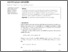# Oscillation criteria for a class of higher odd order neutral difference equations with continuous variable

Wu, Shuhui, Calay, Pargat Singh and Hou, Zhanyuan (2015) Oscillation criteria for a class of higher odd order neutral difference equations with continuous variable. Advances in Difference Equations, 166. pp. 1-17. ISSN 1687-1847Preview
Text
Paper_2_OscillationCriteriaForAClassOf.pdf - Published Version

## Abstract / Description

In this paper, we are mainly concerned with oscillatory behavior of solutions for a class of higher odd order nonlinear neutral difference equations with continuous variable. By converting the above difference equations to the corresponding differential equations and inequalities, the oscillatory criteria are obtained. In addition, examples are given to illustrate the obtained criteria, respectively.

Item Type: Article neutral difference equations; oscillation; continuous variable 500 Natural Sciences and Mathematics > 510 Mathematics School of Computing and Digital Media Bal Virdee 19 Feb 2019 12:25 21 Apr 2020 13:18 https://repository.londonmet.ac.uk/id/eprint/4624View Item